### Showing videos fromEddie Woo with a total of 4,051 videos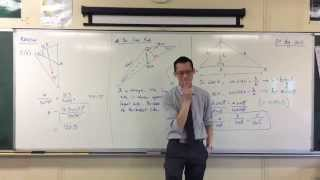10:00
The Sine Rule (2 of 2: Proving & Using the Formula)
3y9m ago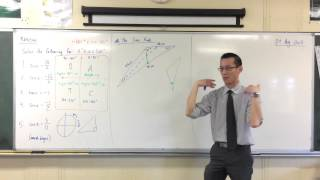07:50
The Sine Rule (1 of 2: What does it actually mean?)
3y9m ago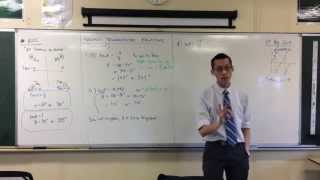05:54
Solving Trigonometric Equations by Quadrants (3 of 3: Why 180°+ x?)
3y9m ago07:46
Solving Trigonometric Equations by Quadrants (2 of 3: Examples)
3y9m ago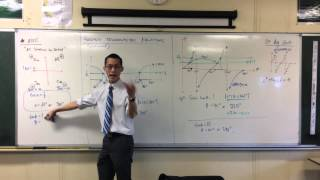04:42
Solving Trigonometric Equations by Quadrants (1 of 3: Introduction)
3y9m ago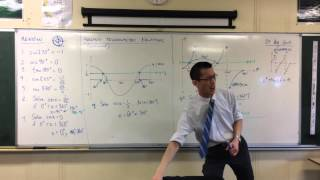05:36
Solving Trigonometric Equations by Graphs (2 of 2: Tan example)
3y9m ago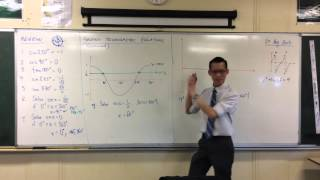04:01
Solving Trigonometric Equations by Graphs (1 of 2: Cosine example)
3y9m ago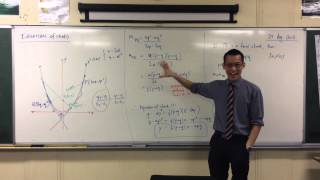09:47
Parametric Equation of Chord (2 of 2: Point-Gradient Form)
3y9m ago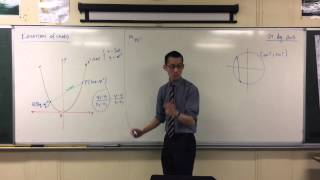08:41
Parametric Equation of Chord (1 of 2: The Gradient)
3y9m ago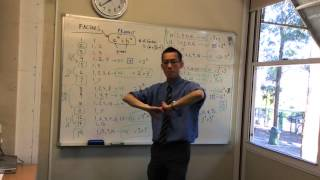13:02
Thinking Deeply About Factors (3 of 3: Finding Explanations)
3y10m ago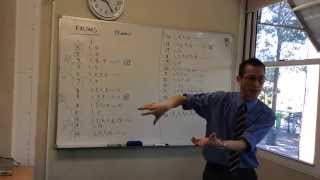05:34
Thinking Deeply About Factors (2 of 3: Strange Patterns)
3y10m ago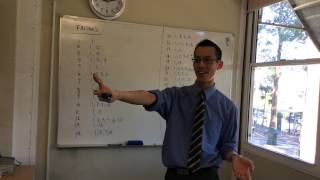10:07
Thinking Deeply About Factors (1 of 3: Initial Observations)
3y10m ago10:17
Solving Trigonometric Equations (2 of 2: Finding the Solutions)
3y10m ago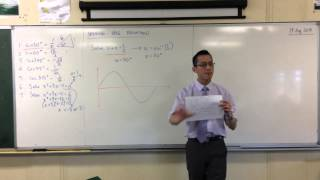09:15
Solving Trigonometric Equations (1 of 2: Thinking Graphically)
3y10m ago10:18
Introduction to Parametrics (3 of 3: A Familiar Example)
3y10m ago08:39
Introduction to Parametrics (2 of 3: What Parameters Actually Are)
3y10m ago09:19
Introduction to Parametrics (1 of 3: Why Parameters Are Useful)
3y10m ago12:08
Evaluating Specific Binomial Coefficients (Exam Questions)
3y10m ago07:25
The Travelling Salesman (3 of 3: Ant Colonisation Heuristic)
3y10m ago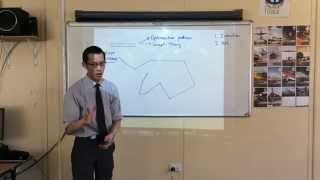11:23
The Travelling Salesman (2 of 3: Nearest Neighbour & SFCs)
3y10m ago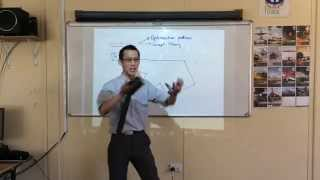07:07
The Travelling Salesman (1 of 3: Understanding the Problem)
3y10m ago09:36
Understanding the graph of tan(x) (2 of 2: Geometric Features)
3y10m ago12:26
Understanding the graph of tan(x) (1 of 2: Thinking Visually)
3y10m ago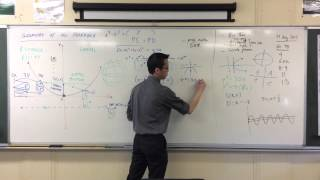04:09
Standard Form of a Parabola (3 of 3: Shifting the Graph)
3y10m ago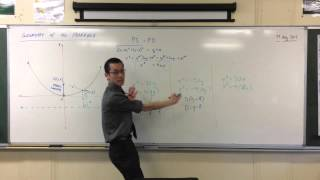06:23
Standard Form of a Parabola (2 of 3: Determining the Focus & Directrix)
3y10m ago08:34
Standard Form of a Parabola (1 of 3: From the Locus Definition)
3y10m ago06:48
Why is there mathematics in STEM? (3 of 3: The Higgs Boson)
3y10m ago12:45
Why is there mathematics in STEM? (2 of 3 Neptune)
3y10m ago12:30
Why is there mathematics in STEM? (1 of 3: Different Kinds of Knowledge)
3y10m ago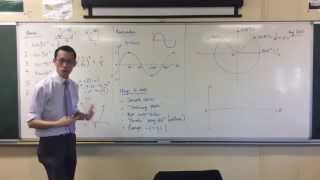08:23
Understanding the Cosine Curve through the Unit Circle
3y10m ago09:53
Space-Filling Curves (4 of 4: Sierpinski Curve)
3y10m ago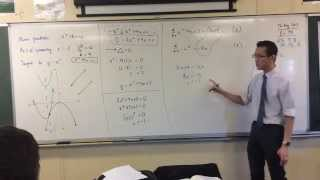11:55
Finding Equations of Parabolas that are Tangent to each other
3y10m ago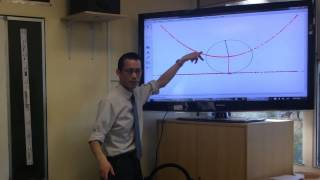10:45
Locus of a Parabola (3 of 3: Animating the Locus)
3y10m ago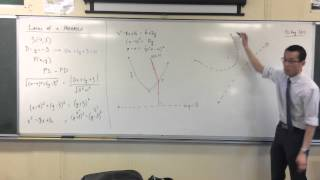03:53
Locus of a Parabola (2 of 3: Understanding the Parabola's Features)
3y10m ago13:12
Locus of a Parabola (1 of 3: Standard Derivation)
3y10m ago07:31
Dimensions (2 of 3: A More Flexible Definition - Scale Factor)
3y10m ago07:09
Dimensions (1 of 3: The Traditional Definition - Directions)
3y10m ago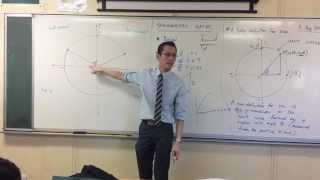12:39
Redefining Sine (3 of 3: Angles Greater Than 90°)
3y10m ago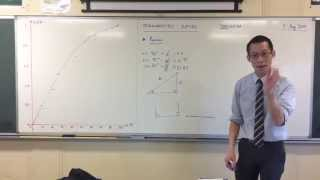10:46
Redefining Sine (1 of 3: Why We Need a Better Definition)
3y10m ago06:21
Introduction to Locus (3 of 3: Alternative Circle Definition)
3y10m ago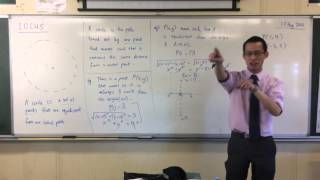06:23
Introduction to Locus (2 of 3: Perpendicular Bisectors)
3y10m ago07:55
Introduction to Locus (1 of 3: Concept & Definition)
3y10m ago05:50
Quadratic Identities (4 of 4: Partial Fractions)
3y10m ago04:53
Quadratic Identities (3 of 4: Comparing Coefficients)
3y10m ago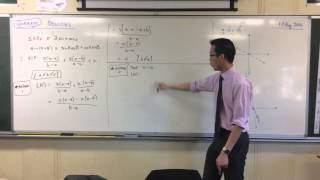10:02
Quadratic Identities (2 of 4: Substituting 3 Values)
3y10m ago06:36
Trigonometric Relationships (3 of 3: Manipulating Sine & Cosine)
3y10m ago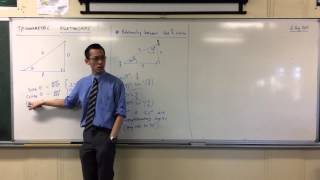11:02
Trigonometric Relationships (2 of 3: Relating Sine & Cosine)
3y10m ago04:22
Trigonometric Relationships (1 of 3: The Basic Ratios)
3y10m ago